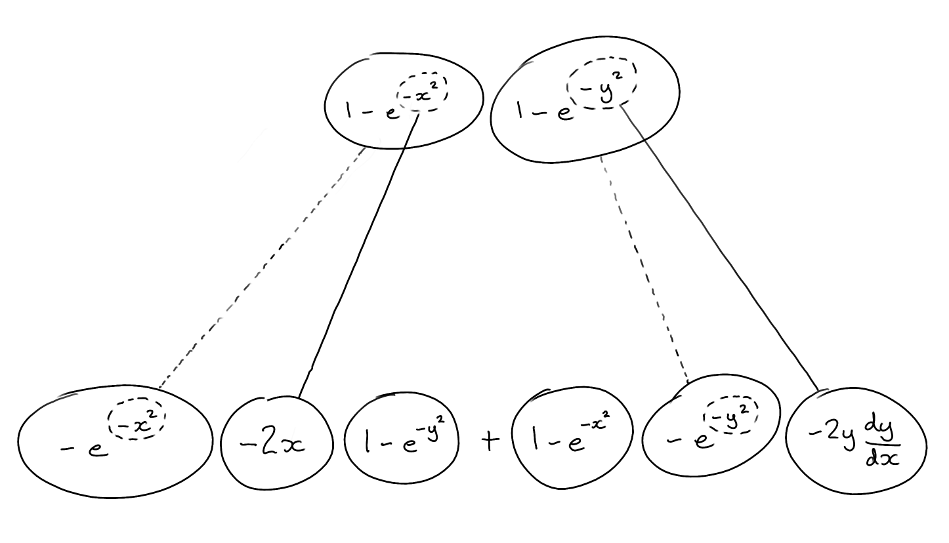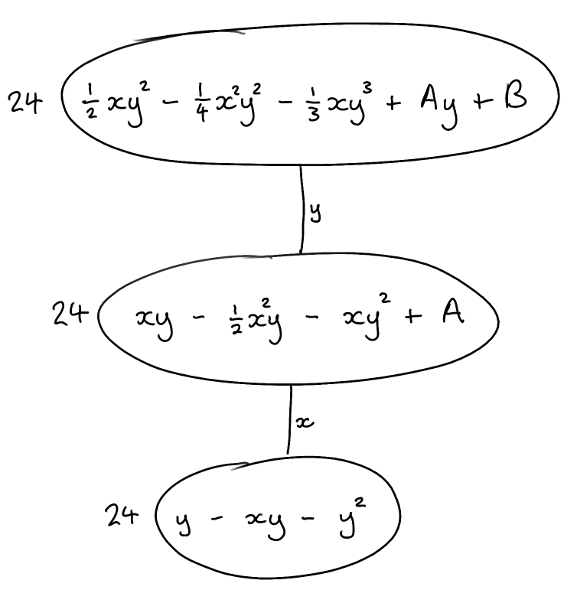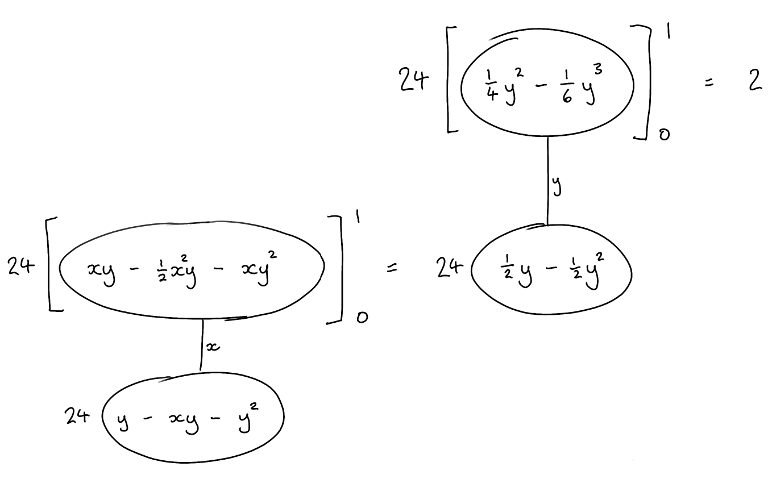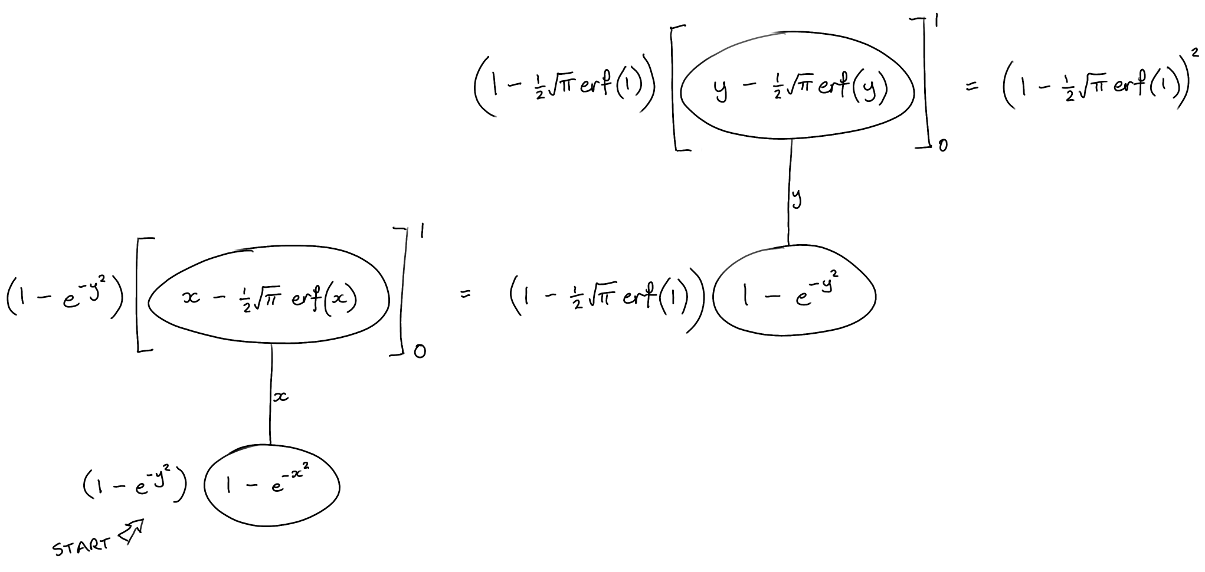# Thread: Integration/Differentiation help.

1. ## Integration/Differentiation help.

Can someone please explain how I would differentiate this:

(1-e^(-x^2))(1-e^(-y^2))

and integrate this:

24y(1-x-y)

Regards.

2. ## Re: Integration/Differentiation help.Originally Posted by Wevans2303Can someone please explain how I would differentiate this:

(1-e^(-x^2))(1-e^(-y^2))

and integrate this:

24y(1-x-y)

Regards.
You need to be more specific.

For the first one are you taking partial derivatives or is y a function of x?

For the integral what are you integrating with respect to? dx, dy, dA, ds ? please clarify.

As asked it is impossible to help you with your questions.

3. ## Re: Integration/Differentiation help.

Apologies TES.

Differentiating w.r.t x and a double integral dydx.

4. ## Re: Integration/Differentiation help.

So for the first question if you have

$\displaystyle f(x,y)=\dfrac{1-e^{-x^2}}{1-e^{-y^2}}$

The partial derivative with respect to x is

$\displaystyle \dfrac{\partial f}{\partial x}=\dfrac{1}{1-e^{-y^2}}\dfrac{\partial }{\partial x} (1-e^{-x^2})= \dfrac{1}{1-e^{-y^2}}(0-e^{-x^2}(-2x))=\dfrac{2xe^{-x^2}}{1-e^{-y^2}}$

For the 2nd one we need to know the domain of integration. What are the limits?

5. ## Re: Integration/Differentiation help.

Oh god i've totally messed this up.

It's the other way round, integrate the first differentiate the second, sorry to have wasted your time.

If you're still in the mood to help, it would be the double integral of 24y(1-x-y ) with limits 0,1 for both x and y.

6. ## Re: Integration/Differentiation help.Originally Posted by TheEmptySetSo for the first question if you have
$\displaystyle f(x,y)=\dfrac{1-e^{-x^2}}{1-e^{-y^2}}$
... whereas, if y is a function of x...... where (key in spoiler) ...

Spoiler:... is the chain rule. Straight continuous lines differentiate downwards (integrate up) with respect to the main variable (in this case x), and the straight dashed line similarly but with respect to the dashed balloon expression (the inner function of the composite which is subject to the chain rule).
But this is wrapped inside each leg of the legs-uncrossed version of...... the product rule, where, again, straight continuous lines are differentiating downwards with respect to x.

The second...I'll maybe change it to use the limits.

Here we are, then...Originally Posted by Wevans2303Oh god i've totally messed this up.

It's the other way round, integrate the first differentiate the second, sorry to have wasted your time.

If you're still in the mood to help, it would be the double integral of 24y(1-x-y ) with limits 0,1 for both x and y.
But that would still be integrating the second?!
__________________________________________________ __________

Don't integrate - balloontegrate!

Balloon Calculus; standard integrals, derivatives and methods

Balloon Calculus Drawing with LaTeX and Asymptote!

7. ## Re: Integration/Differentiation help.Originally Posted by tom@ballooncalculus... whereas, if y is a function of x...... where (key in spoiler) ...

Spoiler:... is the chain rule. Straight continuous lines differentiate downwards (integrate up) with respect to the main variable (in this case x), and the straight dashed line similarly but with respect to the dashed balloon expression (the inner function of the composite which is subject to the chain rule).
But this is wrapped inside each leg of the legs-uncrossed version of...... the product rule, where, again, straight continuous lines are differentiating downwards with respect to x.

The second...I'll maybe change it to use the limits.

Here we are, then...But that would still be integrating the second?!
__________________________________________________ __________

Don't integrate - balloontegrate!

Balloon Calculus; standard integrals, derivatives and methods

Balloon Calculus Drawing with LaTeX and Asymptote!
I'm not making a good first impression here am I!

Sorry, I need help with integrating the first one with limits 0,1 for both x,y and differentiating the second one.

8. ## Re: Integration/Differentiation help.

Ok, and is your first expression a fraction as TES was guessing? Either way, this looks dodgy (i.e. non-elementary, involving the error function).

And in the second, is y a function of x?

9. ## Re: Integration/Differentiation help.Originally Posted by tom@ballooncalculusOk, and is your first expression a fraction as TES was guessing? Either way, this looks dodgy (i.e. non-elementary, involving the error function).

And in the second, is y a function of x?
I need to integrate this (double integration where limits of x are 0,1 and y are 0,1):

f(x,y) = 1-e^(-x^2)* 1-e^(-y^2) for x >0, y>0

I need to differentiate this:

f(x,y) = 24y(1-x-y) x>0,y>0 & x+y<1

That's all I am given in the questions.

10. ## Re: Integration/Differentiation help.Originally Posted by Wevans2303I need to integrate this (double integration where limits of x are 0,1 and y are 0,1):

f(x,y) = 1-e^(-x^2)* 1-e^(-y^2) for x >0, y>0
int 0 to 1 int 0 to 1 &#40;1-e&#94;&#40;-x&#94;2&#41;&#41;&#40;1-e&#94;&#40;-y&#94;2&#41;&#41; dy dx - Wolfram|Alpha

I.e...To differentiate f(x.y) with respect to (e.g.) x, expand and differentiate term by term, treating y as constant.

__________________________________________________ ____________________

Don't integrate - balloontegrate!

Balloon Calculus; standard integrals, derivatives and methods

Balloon Calculus Drawing with LaTeX and Asymptote!

11. ## Re: Integration/Differentiation help.Originally Posted by tom@ballooncalculusint 0 to 1 int 0 to 1 &#40;1-e&#94;&#40;-x&#94;2&#41;&#41;&#40;1-e&#94;&#40;-y&#94;2&#41;&#41; dy dx - Wolfram|Alpha

I.e...To differentiate f(x.y) with respect to (e.g.) x, expand and differentiate term by term, treating y as constant.
Thanks for your help, I think I get it now!

### integration of 24y with respect to x

Click on a term to search for related topics.